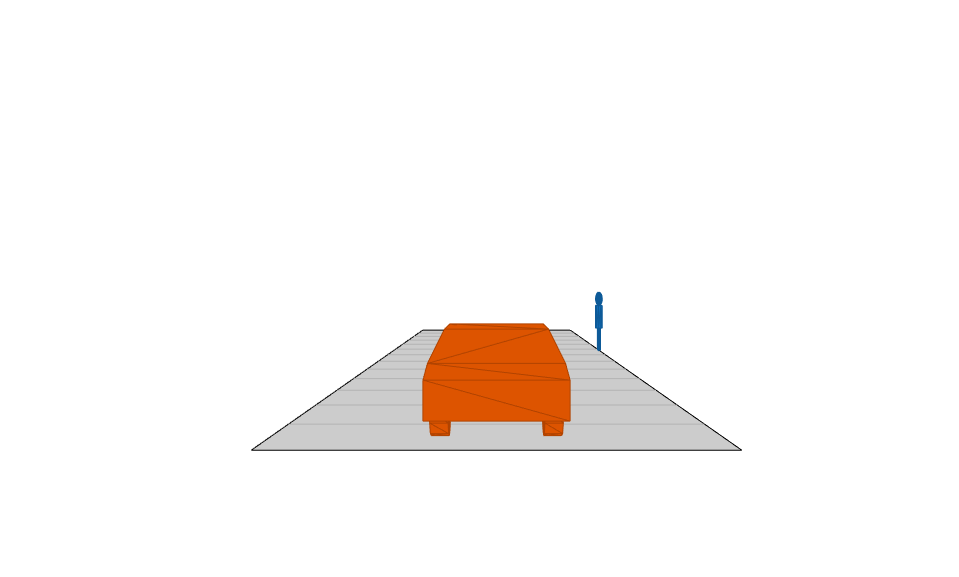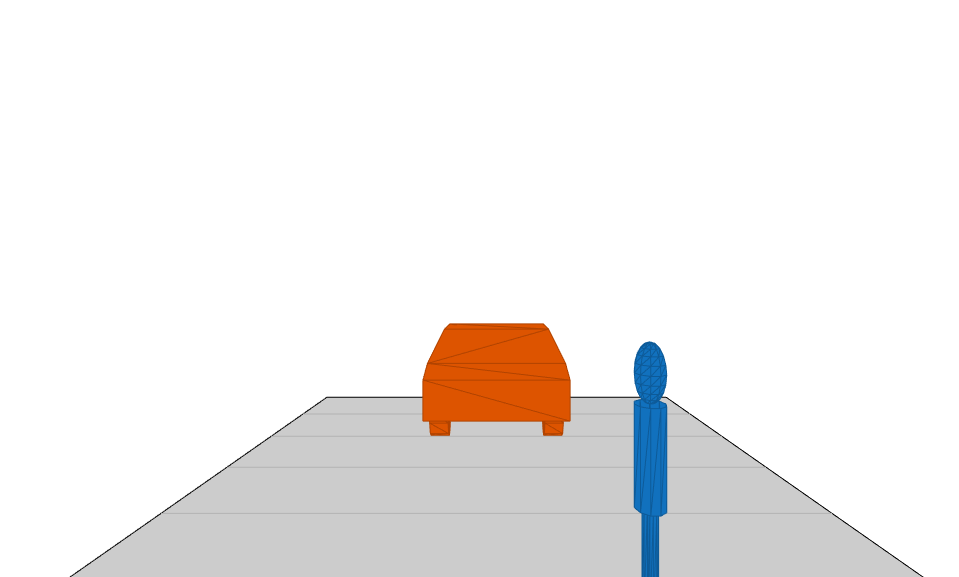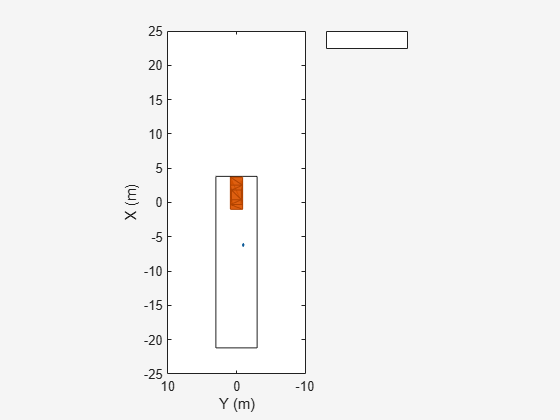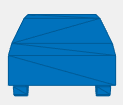# targetMeshes

Mesh vertices and faces relative to specific actor

## Syntax

``[vertices,faces] = targetMeshes(ac)``
``[vertices,faces,colors] = targetMeshes(ac)``

## Description

````[vertices,faces] = targetMeshes(ac)` returns the mesh vertices and faces of all actors in a driving scenario relative to the specified actor, `ac`. When displaying meshes by using a `birdsEyePlot` object, you can use the output mesh information as inputs to the `plotMesh` function.```

example

````[vertices,faces,colors] = targetMeshes(ac)` also returns the color of the mesh faces for each actor.```

## Examples

collapse all

Display actors in a driving scenario by using their mesh representations instead of their cuboid representations.

Create a driving scenario, and add a 25-meter straight road to the scenario.

```scenario = drivingScenario; roadcenters = [0 0 0; 25 0 0]; road(scenario,roadcenters); ```

Add a pedestrian and a vehicle to the scenario. Specify the mesh dimensions of the actors using prebuilt meshes.

• Specify the pedestrian mesh as a `driving.scenario.pedestrianMesh` object.

• Specify the vehicle mesh as a `driving.scenario.carMesh` object.

```p = actor(scenario,'ClassID',4, ... 'Length',0.2,'Width',0.4, ... 'Height',1.7,'Mesh',driving.scenario.pedestrianMesh); v = vehicle(scenario,'ClassID',1, ... 'Mesh',driving.scenario.carMesh); ```

Add trajectories for the pedestrian and vehicle.

• Specify for the pedestrian to cross the road at 1 meter per second.

• Specify for the vehicle to follow the road at 10 meters per second.

```waypointsP = [15 -3 0; 15 3 0]; speedP = 1; smoothTrajectory(p,waypointsP,speedP); wayPointsV = [v.RearOverhang 0 0; (25 - v.Length + v.RearOverhang) 0 0]; speedV = 10; smoothTrajectory(v,wayPointsV,speedV) ```

Add an egocentric plot for the vehicle. Turn the display of meshes on.

```chasePlot(v,'Meshes','on') ```Create a bird's-eye plot in which to display the meshes. Also create a mesh plotter and lane boundary plotter. Then run the simulation loop.

1. Obtain the road boundaries of the road the vehicle is on.

2. Obtain the mesh vertices, faces, and colors of the actor meshes, with positions relative to the vehicle.

3. Plot the road boundaries and actor meshes on the bird's-eye plot.

4. Pause the scenario to allow time for the plots to update. The chase plot updates every time you advance the scenario.

```bep = birdsEyePlot('XLim',[-25 25],'YLim',[-10 10]); mPlotter = meshPlotter(bep); lbPlotter = laneBoundaryPlotter(bep); legend('off') while advance(scenario) rb = roadBoundaries(v); [vertices,faces,colors] = targetMeshes(v); plotLaneBoundary(lbPlotter,rb) plotMesh(mPlotter,vertices,faces,'Color',colors) pause(0.01) end ```## Input Arguments

collapse all

Actor belonging to a `drivingScenario` object, specified as an `Actor` or `Vehicle` object. To create these objects, use the `actor` and `vehicle` functions, respectively.

## Output Arguments

collapse all

Mesh vertices of each actor, returned as an N-element cell array, where N is the number of actors.

Each element in `vertices` must be a V-by-3 real-valued matrix containing the vertices of an actor, where:

• V is the number of vertices.

• Each row defines the 3-D (x,y,z) position of a vertex. The vertex positions are relative to the position of the input actor `ac`. Units are in meters.

Mesh faces of each actor, returned as an N-element cell array, where N is the number of actors.

Each element in `faces` must be an F-by-3 integer-valued matrix containing the faces of an actor, where:

• F is the number of faces.

• Each row defines a triangle of vertex IDs that make up the face. The vertex IDs correspond to row numbers within `vertices`.

Suppose the first face of the `i`th element of `faces` has these vertex IDs.

`faces{i}(1,:)`
```ans = 1 2 3```

In the `i`th element of `vertices`, rows 1, 2, and 3 contain the (x, y, z) positions of the vertices that make up this face.

`vertices{i}(1:3,:)`
```ans = 3.7000 0.9000 0.8574 3.7000 -0.9000 0.8574 3.7000 -0.9000 0.3149```

Color of the mesh faces for each actor, returned as an N-by-3 matrix of RGB triplets. N is the number of actors and is equal to the number of elements in `vertices` and `faces`.

The `i`th row of `colors` is the RGB color value of the faces in the `i`th element of `faces`. The function applies the same color to all mesh faces of an actor.

An RGB triplet is a three-element row vector whose elements specify the intensities of the red, green, and blue components of the color. The intensities must be in the range [0, 1]. For example, `[0.4 0.6 0.7]`.

collapse all

### Meshes

In driving scenarios, a mesh is a triangle-based 3-D representation of an object. Mesh representations of objects are more detailed than the default cuboid (box-shaped) representations of objects. Meshes are useful for generating synthetic point cloud data from a driving scenario.

This table shows the difference between a cuboid representation and a mesh representation of a vehicle in a driving scenario.

CuboidMeshIntroduced in R2020b